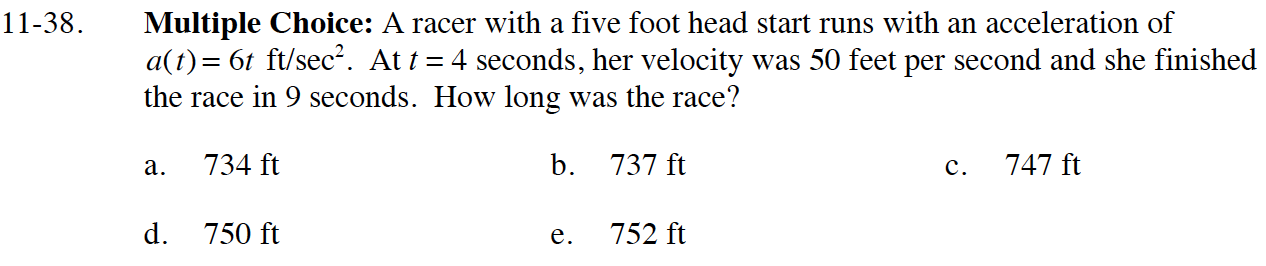### Home > CALC > Chapter 11 > Lesson 11.1.3 > Problem11-38

11-38.Use v(4) = 50 to solve for C.

$d(t)=\int(3t^2+2)dt=t^3+2t+C$

Use d(0) = 5 to solve for C.

Use your equation for d(t) to evaluate d(9).